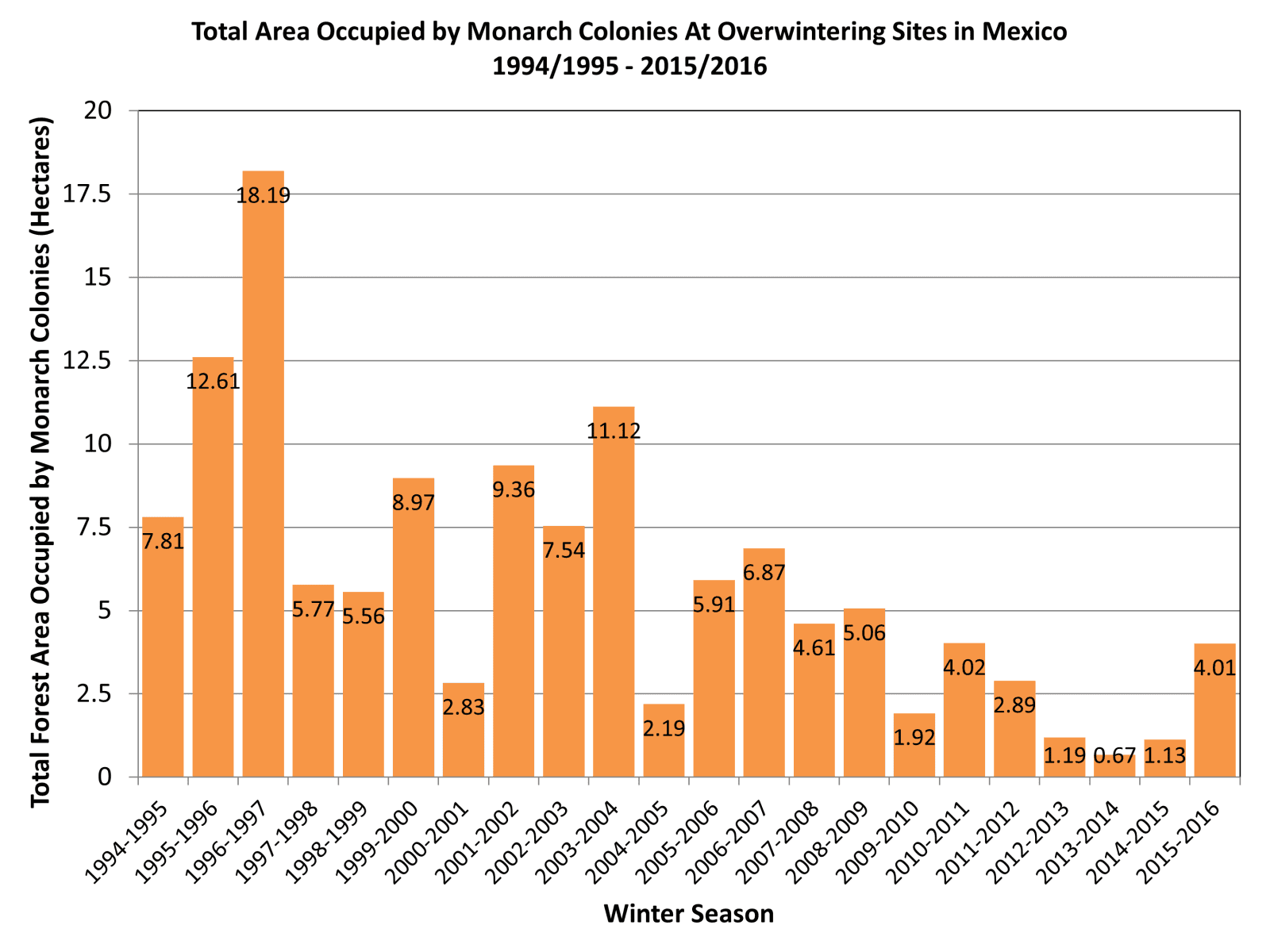Select Page# About This Lesson

This lesson guides students through data entry, representation, analysis, and interpretation. Students will submit data to be used as part of a larger, authentic distributed research project. They will also perform data analysis on the data they collect, and possibly data from other schools and past years; this will help them with their “data jam” that will be incorporated into their citizen science project at the end of the curriculum.

This lesson plan is a component of the EXPLAIN stage of the 5E Learning Model for the overall curriculum.

# Objectives

After this lesson, students will be able to:

• Ask questions and define which questions can be answered with their data.
• Enter data into a spreadsheet.
• Represent data through methods ranging from creating visual graphs to calculating means to performing a linear regression.
• Make predictions about the implications of their results.

## Information for Classroom Use:

Students should know or be familiar with:

• Excel functions
• Terminology from the “Evolutionary Principles” lesson
• Statistical calculations and/or tests ranging from calculating a mean to performing a linear regression, as appropriate for the class

Teachers should know or be familiar with:

• Statistical calculations and/or tests ranging from calculating a mean to performing a linear regression, as appropriate for the class

This lesson is about 50-60 minutes or one class period.

• Computers with Excel
• Lab notebooks/folders